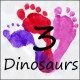# Free Pencil Multiplication Matching Puzzles

Multiplication math facts are something all kids learn. These multiplication matching puzzles are a great way to work on multiplication without multiplication flashcards.

You can match the equation to the answer and have fun learning a the same time.

You work on multiplication from 1 to 12.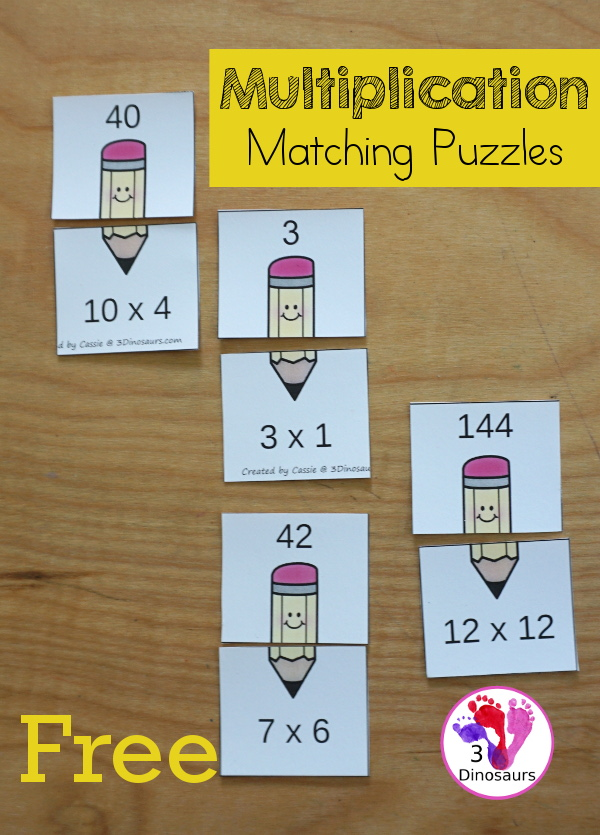We have included links to some of the products and resources we have used with this activity. If you purchase via the links we may earn a small commission.

This is a great addition to the school printables and multiplication printables on the site.

## What is Multiplication?

Multiplication is one of the basic math operations that kids learn.

Multiplication is the adding of equal groups together. So you would be adding groups of 2 together.

You multiply these numbers together to get an answer.

An example is 2 x 3 = 2 + 2 + 2 = 6

These matching multiplication cards work on multiplication 1 to 12 with matching top of pencil multiplication equation with bottom of the pencil with answer.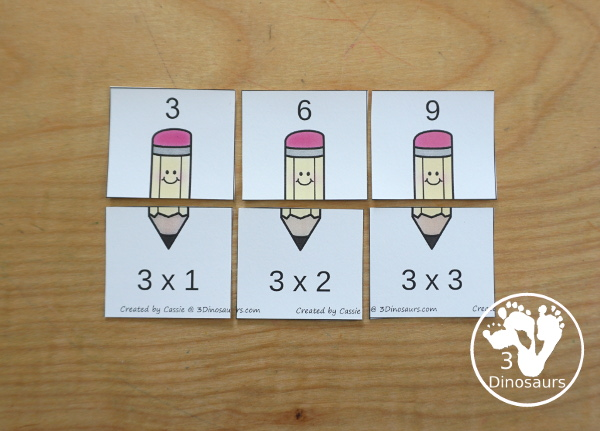## Things to use with the Pencil Multiplication Matching Puzzles Printable

• printer• white cardstock• laminator
• laminating pouches• rings• multiplication bookmarks

## Ways to Use the Pencil Multiplication Matching Puzzles Printable

I’m going to show you a few easy ways to use the multiplication matching printables.

### Matching Multiplication Puzzles from Two Piles

One way of doing the matching activity is to give them two sets of puzzles and have them go through all the pieces and match them. You can see below how setting up the activity might look.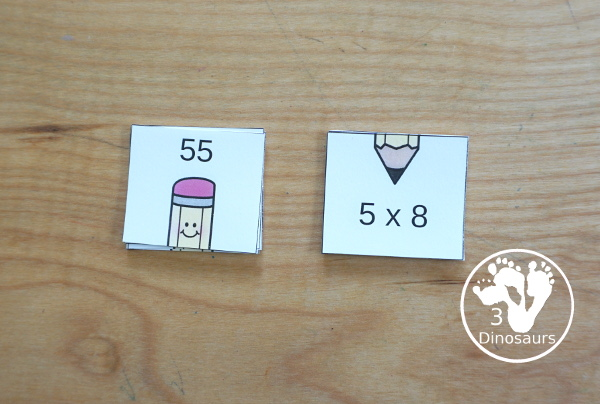### Matching Multiplication Puzzles with Bookmarks

If you are doing this for the first time and need some extra help the multiplication bookmarks are great to flip through and help find the answers. Or just give them one bookmark for the set they are working on.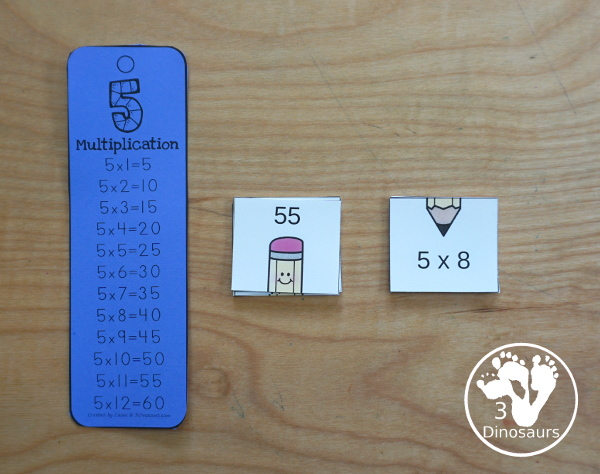### Matching Multiplication Puzzles Sorted

You can sort the top and bottom of the puzzles and lay them on and do a matching game. You can also challenge yourself by turning them over so you can’t see the cards. It is fun to change up how you use the multiplication puzzles.### Matching Multiplication Puzzles on Rings for Center

You can have lots of fun matching multiplication puzzles on rings. This is a great way to match the multiplication equation and answer. Plus it is easy to use and keep trace of in a learning center.## More School Multiplication Printables

If you are looking for more multiplication printables check out the School Theme Math Printables Multiplication & Division. You have 30 days of printables to work on math facts. Plus no-prep printable makes it easy to use.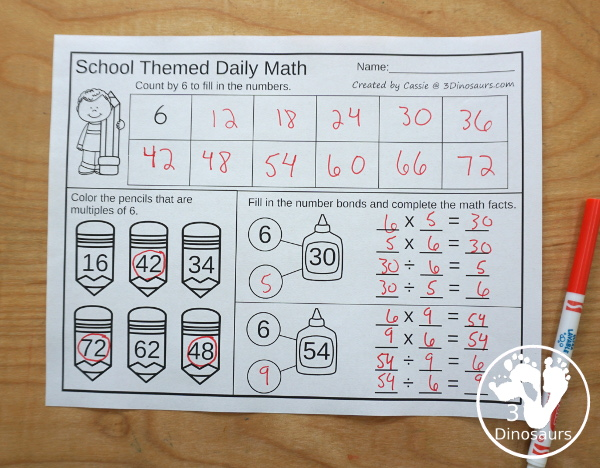## What in the No-Prep School Theme Math Printables Multiplication & Division

It is a collection of 30 pages on no-prep activities for multiplication and division. It also includes great math center activities of roll and graph and a math fact sheet.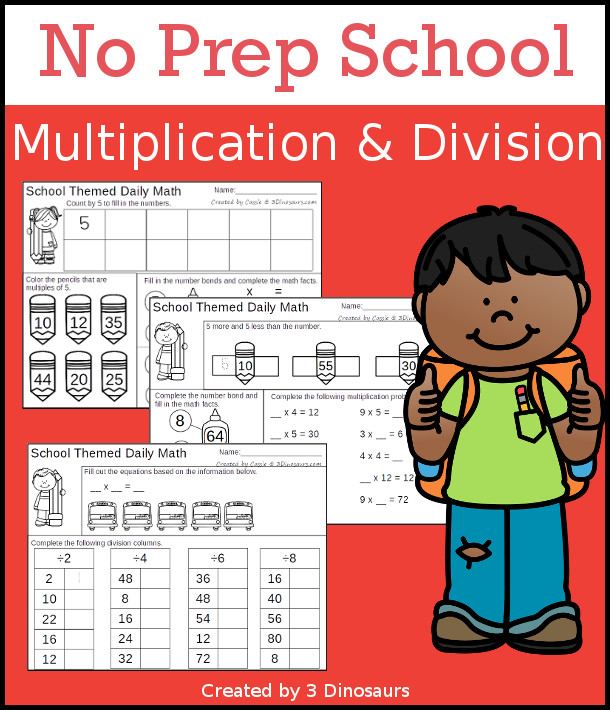### What you will find in the School Theme Math Printables Multiplication & Division:

• 30 pages of no-prep printables for multiplication and division
• School Themed Math Fact Review Page
• Roll & Graph multiplication for 1 to 6 and 1 to 12
• Spinner to use with Roll & Graph and math fact page
• Price: \$4

You can check out a sample of the pages here.

## What you will find in the Free Pencil Multiplication Matching Puzzles

• Multiplication matching Puzzles for numbers 1 to 12
• 1 Number set on each page
• Matching multiplication equation with answer
• 12 pages## Check out these other multiplication printables

Multiplication Flashcards has numbers 1 to 12 for kids to practice their math facts. They come in 5 color options.

Mini Multiplication Flashcards has multiplication from 1 to 12 cards. Plus add on cards for 13 to 15.

Apple Themed Multiplication Cut & Paste is like the beach and has numbers from 1 to 12. You cut and paste the answer on the tree.

School Themed No-Prep Math: Multiplication & Division

Pencil Groups Multiplication Clip Cards has groups from 1 to 12 with multiplication 1 to 12 with a recording sheet.

Check out all the fun Multiplication Printables & Activities on 3 Dinosaurs.

Cassie – 3Dinosaurs.com